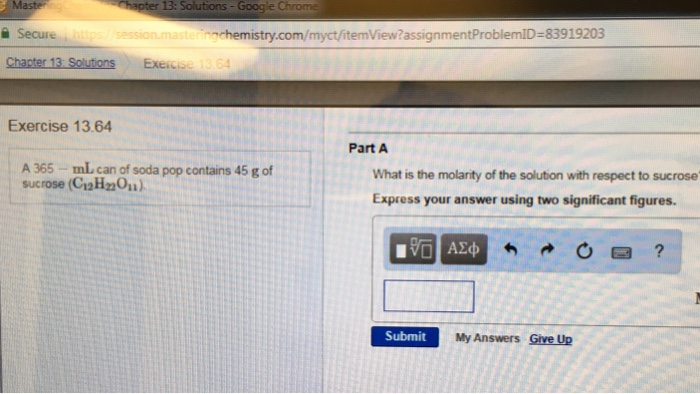# Solution:  A 365 ml can of soda pop contains 45 g of sucrose (C12H22O11) What is the molarity of the solution with respect to sucrose Express your answer using two significant figures.

###### Problem

A 365 ml can of soda pop contains 45 g of sucrose (C12H22O11) What is the molarity of the solution with respect to sucrose Express your answer using two significant figures.View Complete Written Solution